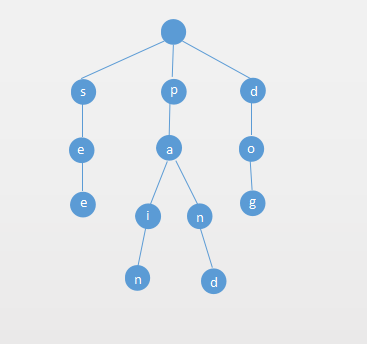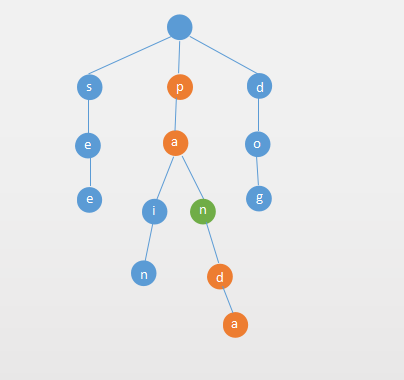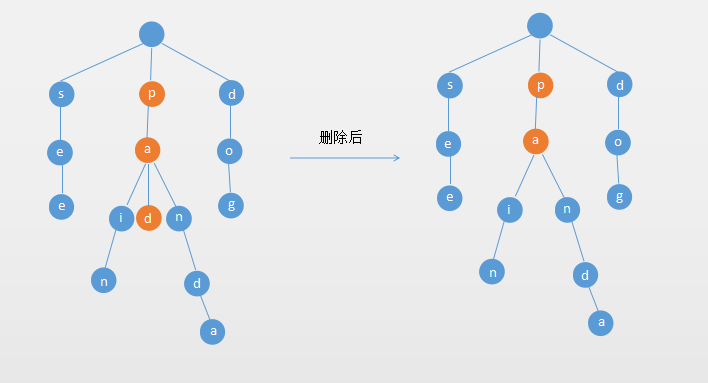# 数据结构与算法（十一）Trie字典树

1. Trie字典树的基本概念
2. Trie字典树的基本操作
1. 插入
2. 查找
3. 前缀查询
4. 删除
3. 基于链表的Trie字典树
4. 基于Trie的Set性能对比
5. LeetCode相关线段树的问题
1. LeetCode第208号问题
2. LeetCode第211号问题
3. LeetCode第677号问题

## Trie字典树的基本概念

Trie字典树主要用于存储字符串，Trie 的每个 Node 保存一个字符。用链表来描述的话，就是一个字符串就是一个链表。每个Node都保存了它的所有子节点。## Trie字典树的基本操作

### 插入

1. 是否是单词 (boolean isWord)
2. 节点所有的子节点，用map来保存 (Map next)

public void add(String word) {
Node current = root;
char[] cs = word.toCharArray();
for (char c : cs) {
Node next = current.next.get(c);
if (next == null) {
//一个字符对应一个Node节点
current.next.put(c, new Node());
}
current = current.next.get(c);
}
//current就是word的最后一个字符的Node

//如果当前的node已经是一个word，则不需要添加
if (!current.isWord) {
size++;
current.isWord = true;
}
}



### 查找

Trie查找操作就比较简单了，遍历带查找的字符串的字符，如果每个节点都存在，并且待查找字符串的最后一个字符对应的Node的 isWord 属性为 true ，则表示该单词存在，伪代码如下：

public boolean contains(String word) {
Node current = root;
for (int i = 0; i < word.length(); i++) {
char c = word.charAt(i);
Node node = current.next.get(c);
if (node == null) {
return false;
}
current = node;
}
//current就是word的最后一个字符的Node
return current.isWord;
}



### 前缀查询

public boolean containsPrefix(String prefix) {
Node current = root;
for (int i = 0; i < prefix.length(); i++) {
char c = prefix.charAt(i);
Node node = current.next.get(c);
if (node == null) {
return false;
}
current = node;
}
return true;
}



### 删除

Trie的删除操作就稍微复杂一些，主要分为以下3种情况：

#### 如果单词是另一个单词的前缀#### 如果单词的所有字母的都没有多个分支，删除整个单词#### 如果单词的除了最后一个字母，其他的字母有多个分支## 基于链表的Trie字典树

public class Trie {

private Node root;

private int size;

private static class Node {
public boolean isWord;
public Map<Character, Node> next;

public Node() {
next = new TreeMap<>();
}

public Node(boolean isWord) {
this();
this.isWord = isWord;
}

}

public Trie() {
root = new Node();
}

public int size() {
return size;
}

public boolean isEmpty() {
return size == 0;
}

/**
* 插入操作
*
* @param word 单词
*/
Node current = root;
char[] cs = word.toCharArray();
for (char c : cs) {
Node next = current.next.get(c);
if (next == null) {
current.next.put(c, new Node());
}
current = current.next.get(c);
}
//如果当前的node已经是一个word，则不需要添加
if (!current.isWord) {
size++;
current.isWord = true;
}
}

/**
* 是否包含某个单词
*
* @param word 单词
* @return 存在返回true，反之false
*/
public boolean contains(String word) {
Node current = root;
for (int i = 0; i < word.length(); i++) {
char c = word.charAt(i);
Node node = current.next.get(c);
if (node == null) {
return false;
}
current = node;
}
//如果只存在 panda这个词，查询 pan，虽然有这3个字母，但是并不存在该单词
return current.isWord;
}

/**
* Trie是否包含某个前缀
*
* @param prefix 前缀
* @return
*/
public boolean containsPrefix(String prefix) {
Node current = root;
for (int i = 0; i < prefix.length(); i++) {
char c = prefix.charAt(i);
Node node = current.next.get(c);
if (node == null) {
return false;
}
current = node;
}
return true;
}

/*
* 1，如果单词是另一个单词的前缀，只需要把该word的最后一个节点的isWord的改成false
* 2，如果单词的所有字母的都没有多个分支，删除整个单词
* 3，如果单词的除了最后一个字母，其他的字母有多个分支，
*/

/**
* 删除操作
*
* @param word
* @return
*/
public boolean remove(String word) {
Node multiChildNode = null;
int multiChildNodeIndex = -1;
Node current = root;
for (int i = 0; i < word.length(); i++) {
Node child = current.next.get(word.charAt(i));
//如果Trie中没有这个单词
if (child == null) {
return false;
}
//当前节点的子节点大于1个
if (child.next.size() > 1) {
multiChildNodeIndex = i;
multiChildNode = child;
}
current = child;
}
//如果单词后面还有子节点
if (current.next.size() > 0) {
if (current.isWord) {
current.isWord = false;
size--;
return true;
}
//不存在该单词，该单词只是前缀
return false;
}
//如果单词的所有字母的都没有多个分支，删除整个单词
if (multiChildNodeIndex == -1) {
root.next.remove(word.charAt(0));
size--;
return true;
}
//如果单词的除了最后一个字母，其他的字母有分支
if (multiChildNodeIndex != word.length() - 1) {
multiChildNode.next.remove(word.charAt(multiChildNodeIndex + 1));
size--;
return true;
}
return false;
}
}



## 基于Trie的Set性能对比

Pride and Prejudice
Total words: 125901
Total different words: 6530

TrieSet       Time: 0.099788784
BSTSet        Time: 0.339963625


A Tale of Two Cities
Total words: 141489
Total different words: 9944

TrieSet       Time: 0.119505174
BSTSet        Time: 0.331334495


War and Peace
Total words: 602359
Total different words: 16725

TrieSet       Time: 0.09750872
BSTSet        Time: 0.233328074


## 更多关于Trie的话题

public Node() {
next = new HashMap<>();
}


public Node(boolean isWord){
this.isWord = isWord;
next = new Node;//只能存储26个小写字母
}


Trie查询效率非常高，但是对空间的消耗还是挺大的，这也是典型的空间换时间。

## LeetCode相关线段树的问题

#### LeetCode第208号问题

Trie trie = new Trie();

trie.insert("apple");
trie.search("apple");   // 返回 true
trie.search("app");     // 返回 false
trie.startsWith("app"); // 返回 true
trie.insert("app");
trie.search("app");     // 返回 true


#### LeetCode第211号问题

void addWord(word)
bool search(word)
search(word)


addWord("bad")
search("b..") -> true


public boolean searchByWildCard(String express) {
return search(root, express, 0);
}

private boolean search(Node node, String express, int index) {
//如果已经到了待查询字符串的尾端了
if (index == express.length()) {
return node.isWord;
}
char c = express.charAt(index);
if (c != '.') {
Node nextChar = node.next.get(c);
if (nextChar == null) {
return false;
}
return search(nextChar, express, index + 1);
} else {//如果是通配符
Map<Character, Node> nextNodes = node.next;
//遍历所有的子节点
for (Map.Entry<Character, Node> entry : nextNodes.entrySet()) {
if (search(entry.getValue(), express, index + 1)) {
return true;
}
}
return false;
}
}



#### LeetCode第677号问题

输入: insert("apple", 3), 输出: Null



class Node {
public int value;
public Map<Character, Node> next;
}

public int sum(String prefix) {
Node cur = root;
for (int i = 0; i < prefix.length(); i++) {
char c = prefix.charAt(i);
Node node = cur.next.get(c);
if (node == null) {
return 0;
}
cur = node;
}

//cur指向prefix的最后一个字符的Node

//对每个以prefix为前缀的node进行累加
return countValue(cur);
}

private int countValue(Node node) {
int result = node.value;
for (char c : node.next.keySet()) {
result += countValue(node.next.get(c));
}
return result;
}



## Reference03-277421
08-2947311-2594
08-244万+
09-107万+
01-02301
07-10377
05-172030
11-163万+
05-09133